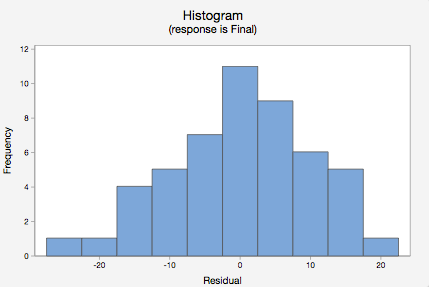# 12.3.3 - Minitab Express - Simple Linear Regression

## MinitabExpress – Obtaining Simple Linear Regression Output

We previously created a scatterplot of quiz averages and final exam scores and observed a linear relationship. Here, we will use quiz scores to predict final exam scores.

1. Open the data set:
2. On a PC or Mac: Select STATISTICS > Regression > Simple Regression
3. Double click Final in the box on the left to insert it into the Response (Y) box on the right
4. Double click Quiz_Average in the box on the left to insert it into the Predictor (X) box on the right
5. Under the Graphs tab, click the box for Residual plots
6. Click OK

This should result in the following output:

Simple Regression: Final versus Quiz_Average
Analysis of Variance
Regression 1 2663.66 2663.66 28.24 <0.0001
Error 48 4527.06 94.31
Total 49 7190.72
Model Summary
9.71152 37.04% 35.73%
Coefficients
Term Coef SE Coef T-Value P-Value
Constant 12.12 11.94 1.01 0.3153
Quiz_Average 0.7513 0.1414 5.31 <0.0001
 Final = 12.12 + 0.7513 Quiz_Average
 Obs Final Fit Resid Std Resid 11 49 70.4975 -21.4975 -2.25 R 40 80 61.2158 18.7842 2.03 R 47 37 59.5050 -22.5050 -2.46 R

R Large residualVideo Walkthrough

Select your operating system below to see a step-by-step guide for this example.

On the next page you will learn how to test for the statistical significance of the slope.